## Pages

Showing posts with label exponential. Show all posts
Showing posts with label exponential. Show all posts

### Exponential Growth and Decay

In this section we will solve typical word problems that involve exponential growth or decay.
If k is positive then we will have a growth model and if k is negative then we will have a decay model.

Use the exponential growth/decay model to answer the questions.

Example: A certain bacterium has an exponential growth rate of 25% per day.  If we start with 0.5 gram and provide unlimited resources how much bacteria can we grow in 2 weeks?
Answer: We can grow 16.56 grams of bacteria in 2 weeks time.

Typically the exponential growth rate will not be given.  In this case, we must determine that before we can use the model to answer the question.

Step 1: Use the given information to calculate the growth/decay rate k.
Step 2: Substitute the initial amount and k to formulate a model.
Step 3: Us the model to answer the question.

Example: During its exponential growth phase, a certain bacterium can grow from 5,000 cells to 12,000 cells in 10 hours.  At this rate how many cells will be present after 36 hours?
Answer: About 116,877 cells in 36 hours time.

Tip: Use the exact value for k and avoid round-off error.  If we use the approximate rounded-off value for k we will compound the error by rounding off again at the end when calculating the final result.

Example: During its exponential growth phase, a certain bacterium can grow from 5,000 cells to 12,000 cells in 10 hours.  At this rate how long will it take to grow to 50,000 cells?
Answer: It will take approximately 26.3 hours.

Example: A certain animal species can double its population every 30 years. Assuming exponential growth, how long will it take the population to grow from 40 specimens to 500?
Answer: it will take about 109.3 years.

Up to this point, we have seen only exponential growth.  We will conclude this section with some exponential decay applications.  Often exponential rate of decay can be determined from the half-life information.  Half-life is the amount of time it takes for a substance to decay to half of the original amount.

Example: A certain isotope has a half-life of 4.2 days. How long will it take a 150-milligram sample to decay so that only 10 milligrams are left?
Answer: It will take about 16.4 days.

Example: The half-life of carbon-14 is 5730 years. If it is determined that an old bone contains 85% of it original carbon-14 how old is the bone?
Answer: It takes about 1,343.5 years for a bone to lose 15% of its carbon-14.

To summarize, first find the growth/decay rate. Put together a mathematical model using the initial amount and the exponential rate of growth/decay.  Then use the model to answer the question.

YouTube Video:

---

### Intermediate Algebra Exam #4

Click on the 10 question exam covering topics in chapter 7. Give yourself one hour to try all of the problems and then come back and check your answers.

IA Sample Exam #4 ]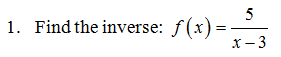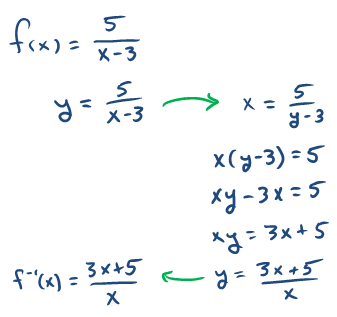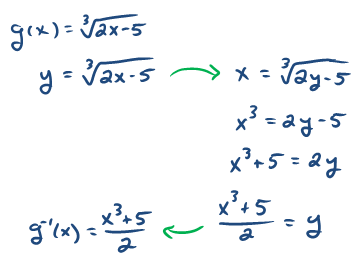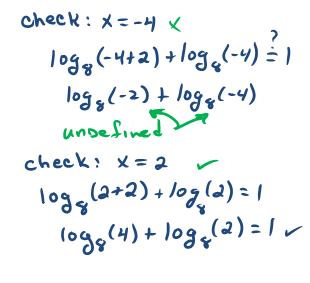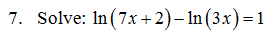8. A \$1,000 investment is made at an annual interest rate of  7 3/4% that is compounded quarterly.  How long will it take the investment to double?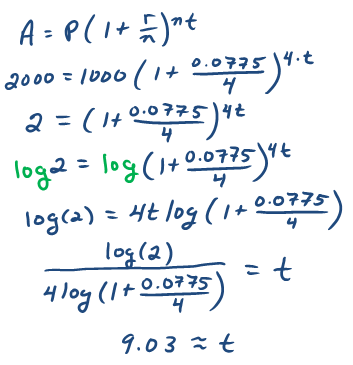9. Francium-223 has a half-life of about 22 minutes. How much of a 3-microgram sample of francium-223 will be left after 5 minutes of decay? Round off answer to the nearest hundredth of a microgram.10. In the year 2000 a certain small town had a population of 72,000 people.  In the year 2010 the population was estimated to have grown to 108,000 people. If the population continues to grow exponentially at this rate, estimate the population in the year 2016.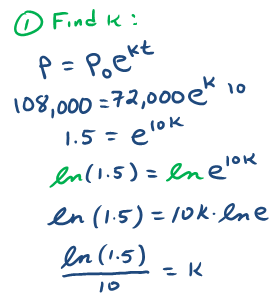---